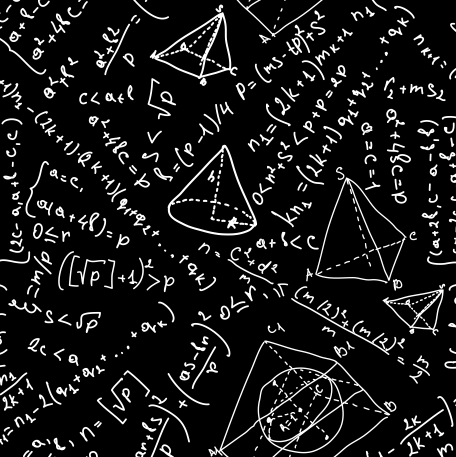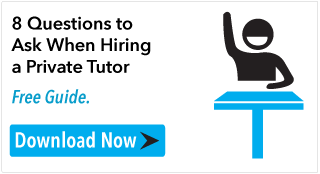# ACT & SAT Prep and College Admissions BlogThe ACT Math section tests math skills that every high school student should have.  Even if you think you are good at math, it won’t be a walk in the park, and even if you think you are bad at math, it won’t be impossible.  It will test not just what you already know, but your ability to figure things out on the fly, so memorization and practice are both a necessary part of the preparation process.

### Format

While the other multiple choice sections on the ACT have four answer choices, the questions in the ACT Math section have five.  You are allowed sixty minutes to answer sixty questions, but this doesn’t mean that each question will take a minute to answer.  The questions span a range of difficulties, so some you will be able to answer quickly while others will take longer

### Instructions

While each version of the ACT will have different questions (you won’t see the same questions if you take the test more than once), the instructions are always identical.  On test day you should already know the instructions for each section by heart so you don’t have to spend time reading them.  These are the instructions for the Math section:

“After solving each problem, pick the correct answer from the five given and fill in the corresponding oval on your answer sheet. Solve as many problems as you can in the time allowed. Do not worry over problems that take too much time; skip them if necessary and return to them if you have time.

Calculator use is permitted on the test. Calculators can be used for any problem on the test, though calculators may be more harm than help for some questions.

Note: unless otherwise stated on the test, you should assume that:

1. Figures accompanying questions are not drawn to scale.

2. Geometric figures exist in a plane.

3. When given in a question, “line” refers to a straight line.

4. When given in a question, “average” refers to the arithmetic mean.”

### Problem Types

There are two types of math problems on the ACT, Basic Problems and Word Problems.  Basic problems are written out in mathematical symbols and equations and are self-explanatory.  Word problems are written in words and sentences.  In order to solve word problems you have to be able to translate them into mathematical formulas.

### Subjects

The content of the problems in the Math section is taken from the following subjects:

• Pre-Algebra
• Elementary Algebra
• Intermediate Algebra
• Coordinate Geometry
• Plane Geometry
• Trigonometry

Note that AP subjects like calculus and statistics are not included.  Just because you have taken more advanced math classes doesn’t necessarily mean that you will have an edge on this part of the exam over someone who hasn’t.

Dane Dormio is an online tutor and blogger with an unconventional approach to education.  Visit his tutoring blog at www.synergy-tutoring.com.